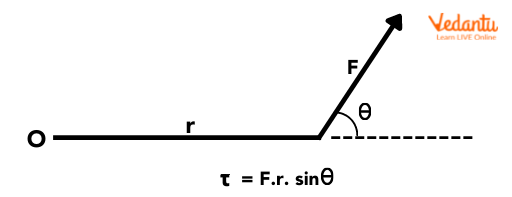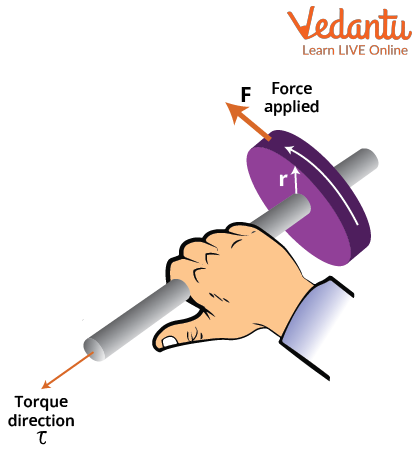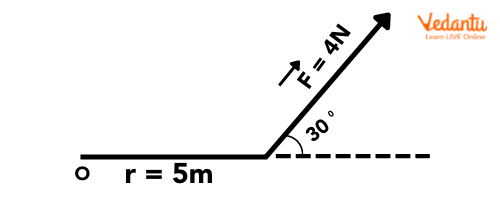Courses
Courses for Kids
Free study material
Free LIVE classes
More

# Torque and Its Type - Numerical Problems and Real Life ExamplesLIVE
Join Vedantu’s FREE Mastercalss

## Torque - Rotational Variant of Linear Force

Torque is basically the rotating force acting on any object or body. It can be termed as the rotational variant of linear force. Just like a linear force causes an acceleration of the body, a torque leads to the angular acceleration. A very popular example of torque is mostly given by considering a door. When we apply force on a door to open it, we’re basically applying a torque on it. We cause the door to rotate about the hinges.

Torque depends on the distance between the axis of rotation and the point where the force is applied. Again coming back to our example of the door, it is noticeable that the handle of the door is kept as far away from the hinge as possible. This is due to the fact that the torque depends on the distance between the point of application of the force and the axis of rotation. If you apply force close to the hinges you will notice that a lot more effort is required to open the door than when you use the handle. The torque definition essentially refers to the “tendency of force to rotate the body to which it is applied’.

## What is Torque?

We have discussed torque in general terms, but now we’ll define the term considering the Physics behind it. So torque can be defined as the force that can cause an object to rotate along an axis is measured as torque. Similar to how force accelerates an object in linear kinematics, torque accelerates an object in an angular direction. In simple terms, we can now state that angular acceleration is the effect of the resulting torque.

Mathematically, torque can be defined as the vector product of the force applied and the distance between the point of application of the force and the axis of rotation. Since a vector product is used, we can infer that torque is a vector quantity, and it acts perpendicular to the plane of the force and the radius at which the force acts with respect to the axis of rotation. The torque produced by a force will be different for different axes of rotation.Let us assume a force F is applied on an object which in turn rotates it thereby giving rise to a torque. This can be mathematically represented as,

\begin{align} &\tau=F \times R \\ &\tau=F \cdot R \sin \theta \end{align}

where R is the distance between the point of application of the force F and the pivot point of the object and θ is the angle between F and the axis of the object. The above equation is known as the torque equation.

As torque is a product of force and distance, the S.I. unit of torque is Newton-Meter (N-m). It has dimensions ML2T-2Torque is usually represented by the Greek letter tau $\tau$ and is known as torque symbol.

### Direction of Torque

Torque is just the vector or cross product of the force and the radius at which the force acts from the pivot point or the axis of rotation. Hence, the direction of the torque applied can be found by using the right-hand thumb rule where we curl our fingers in the direction pointing towards the force. The thumb will then point towards the direction of the torque vector. The torque acts perpendicular to the plane of the force and radius as we have already stated before. The diagram below will make this clear.Direction of torque using right-hand thumb rule

## Types of Torque

The torque applied on any object can be of two types. The torque is differentiated based on its ability to produce an angular acceleration. It can either produce an angular acceleration or it cannot produce an angular acceleration. This way torque is divided into two types.

(1) Static Torque: The type of torque that does not result in angular acceleration of the item on which the force is being applied to, is termed as static torque.

An example of static torque would be when a force is applied on a see-saw which is present in a ground, it will not rotate and doesn't experience any angular acceleration.

(2) Dynamic Torque: The type of torque that actually can produce angular acceleration is termed as dynamic torque.

Dynamic torque is exhibited in shaft rotation in motors. Dynamic torque causes a change in rotational motion of the shaft.

## Numerical Examples of Torque:

Example 1: Find the torque produced at point O in the below figure,when the force is 4 N and the lever arm is 5m in length.Diagram for Example 1

Solution: Let us consider the above Image.

As we know, Torque, $\tau =F\cdot r\sin \theta$. (Here, F is the force vector and r is the length of the lever arm. $\theta$ is the angle between $\overrightarrow{F}$ and r)

From the figure, we can see that, F=4N, r=5m and $\theta =30{}^\circ$

$\therefore \tau \,=\,\,5\times 4\times \sin 30$

or, $\tau =10\,N-m$

As we can see from the above given example, the torque produced at the point O is equal to 10 N-m.

Example 2: Find the torque acting on a body of mass 5kg having an acceleration of the is 10 $\dfrac{cm}{{s}^{2}}$.

The length of the lever arm is $4\,m$ and the angle between the force vector and the plane is ${{60}^{\circ}}$.

Solution: To calculate torque, we must find out first the force the body can fundamentally apply.

As you already know, Force $F=m\times a$, where m is the mass of the body and a is the acceleration.

$\therefore F=m\times a$

Or, $F=(5)\times 10$

$\therefore F=50\,N$ (in CGS units)

Therefore, the required torque, $\tau$ produced by the body is given as follows:

$\tau =F\cdot r\sin \theta$

Or, $\tau =50\times 4\sin {{60}^{o}}=-\,60.96\,N-m$

This means that the direction of the torque is opposite to that of the direction of the force vector.

## Conclusion

The rotating tendency that can be observed when force is applied to any object around its axis is termed as torque. The magnitude of torque is calculated as the cross product between the force applied and the shortest distance between the axis of rotation and the point of application of the force. This distance is also referred to as the length of the lever arm.

The mathematical formula of torque is given by, $\tau =F\cdot r\sin \theta$, where $\tau$ is the torque, F is the magnitude of the force applied, r is the length of the lever arm and $\theta$ is the angle between F and r. The S.I. unit of torque is Newton-meter(N-m).

The direction of torque can be found by using the right hand thumb rule. Torque can be either dynamic or static in nature depending on whether it can or cannot produce an angular acceleration respectively.

Last updated date: 24th Sep 2023
Total views: 135k
Views today: 1.35k

## FAQs on Torque and Its Type - Numerical Problems and Real Life Examples

1. What are some real-life examples of torque?

One of the most common examples of torque that we see on a daily basis is the opening and closing of doors. We can also observe in opening the lid of a jar and maneuvering the steering wheel. We can see this really applies when we play a see-saw anytime. When we are riding a bike, the force acting on the wheel results in a torque which is responsible for the rotation of the wheel. In gyroscopes and pendulums, the torque equation is used extensively.

2. How is torque and speed related in cars?

Torque is basically the rotating motion produced by the force applied on the body. In automobiles and cars, when the engine produces maximum torque, the output is also maximized, resulting in the maximum speed at which the car can move. Race cars increase the output by maximizing the torque produced. Basically, torque is inversely proportional to speed since as output speed is increasing, then the working torque is reduced proportionately. The fastest cars can produce higher levels of torque at low rpm speed.